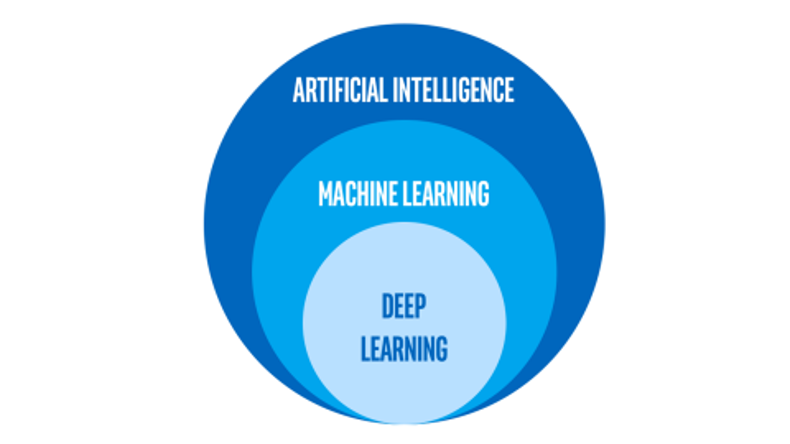A Brief Introduction to Machine LearningIntroduction

What exactly is machine learning?

• Conversationally, it depends on who you ask:
• Other software engineers: the latest craze in software development
• Laborers: a new technology looking to take their jobs
• Media: a dangerous technology bent on taking over the world

Today, we will be discussing:

• Formal definition of machine learning and related topics
• Common types of machine learning projects
Hierarchy of Artificial Intelligence

Artificial Intelligence

• Any technique which enables computers to mimic human behavior

Machine Learning

• AI techniques that give computers the ability to learn without being explicitly programmed to do so

Deep Learning

• A subset of ML will make the computation of multi-layer neural networks feasible
Hierarchy of Artificial Intelligence, cont.Classification vs. Regression

Classifications:

• Calculations are made to predict where a particular outcome will be placed in a finite number of categories based on given parameters
• Ex: Our project will either predict that it will rain or it will not rain tomorrow, there is no in-between. (Drizzling would count as raining) Regression
• Calculations are made to predict a real number outcome based on given parameters
• Ex: Our project could predict it will rain 0 inches tonight. Or it could predict it will rain 1.4 inches tonight. Or it could predict any other real number [0, positive infinity)Mathematical Representation

How does the machine understand these relationships?

• Think back to 9th-grade algebra: y = mx + b
• y: the resulting value (the value we are interested in)
• m: the slope of the prediction line
• x: some input variable that (hopefully) assists in making predictions
• b: the y-intercept (where the line crosses the y-axis)

The machine uses this line to plug in potential x values to attempt to predict the result (the y-value)

Discovering the Relationship

How does the machine know the formula?

• If we simply provided the formula, the machine would not be learning on its own: therefore, it would not be a machine learning project.
• Uses many different strategies: the most popular being gradient descent

Disagree: Computers are stupid but they are stupid very quickly.

Gradient descent uses this mindset and makes poor choices very quickly.

Start by making a “blanket” predictive model.

• Start every value for m and b at 0.
• Start every value for m and b at a random value.

Make small adjustments repeatedly very quickly.

• Usually uses derivatives to assist in determining what adjustment needs to be made.
• Simply: a computerized version of the “hot vs. cold” game.

• The pre-approved number of adjustments have been completed
• The error of the predictive model is considered acceptable
Error? How is that calculated?

The project starts with the “answer key”

• The machine knows what the actual values we are predicting should
• This is called the training data

• Mean Squared Error
Acceptable Error?

No predictive model is likely to be 100% accurate

• Depending on the importance of the project and the time allowed to complete the project, we must accept some other percentage of accuracy

Error is considered acceptable when either

• The error is below a certain threshold
• The error has changed below a certain amount in a certain number of adjustments
Multiple Inputs

Each of our examples so far has had one input and one output

• Most machine learning problems will not be this simple
• y = m1*x1 + m2*x2 + … + mn*xn + b
• Difficult for humans to understand by looking at a graph
• Computers do not care. This is no more confusing to a computer than a problem with a single input.
Machine Learning Pitfalls

Underfitting

• Not providing enough training data for our predictive model to be able to infer any real relationship
• A data set of two houses is not enough to make a predictive model of housing prices

Overfitting

• Harder to notice
• Including irrelevant data
• The color of a house’s front door probably does not greatly affect the price of the house
Bloom’s Taxonomy

Knowledge

• involved the recall of specifics and universals; the recall of methods and processes, or the recall of a pattern, structure, or setting

Comprehension

• refers to a type of understanding or apprehension such that the individual knows what is being communicated and can make use of the material or idea being communicated without necessarily relating it to other material or seeing its fullest implications
Bloom’s Taxonomy

Application

• refers to the use of abstractions in particular and concrete solutions

Analysis

• represents the breakdown of a communication into its constituent elements or parts such that the relative hierarchy of ideas is made clear and/or the relations between ideas expressed are made explicit
Bloom’s Taxonomy

Synthesis

• involves the putting together of elements and parts so as to form a whole

Evaluation

• engenders judgments about the value of material and methods for a given purpose
Sources

1: Oracle Blog Article: What's the Difference Between AI, Machine Learning, and Deep Learning?

2: intel.la Image: AI, Machine Learning and Deep Learning

3: Wikimedia Commons Image: Linear Regression

4: Wikipedia Article: Mean squared error

5: Amazon Tutorial: Model Fit: Underfitting vs. Overfitting

6: Vanderbilt University: Bloom’s Taxonomy